# SAT Math Multiple Choice Question 528: Answer and Explanation

### Test Information

Question: 528

3. A microbiologist placed a bacteria sample containing approximately 2,000 microbes in a petri dish. For the first 7 days, the number of microbes in the dish tripled every 24 hours. If n represents the number of microbes after h hours, then which of the following equations is the best model for the data during the 7-day period?

• A.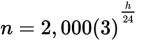• B.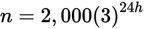• C.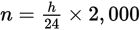• D.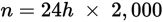Explanation:

A

Difficulty: Medium

Category: Passport to Advanced Math / Scatterplots

Strategic Advice: When the dependent variable in a relationship increases by a scale factor, like doubling, tripling, etc., there is an exponential relationship between the variables which can be written in the form y = a(b)x, where a is the initial amount, b is the scale factor, and x is time.

Getting to the Answer: The question states that the number of microbes tripled every 24 hours, so the relationship is exponential. This means you can eliminate C and D right away. Choices (A) and B are written in the form y = a(b)x, with the initial amount equal to 2,000 and the scale factor equal to 3, so you can't eliminate either one at first glance. To choose between them, try an easy number for h (like 24) in each equation to see which one matches the information given in the question. In the first equation,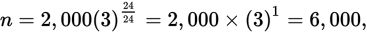which is 2,000 tripled, so (A) is correct.